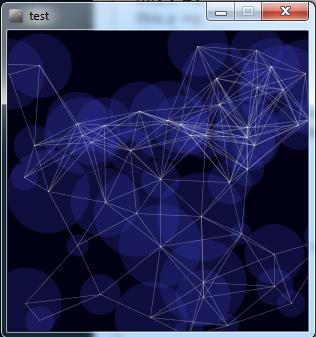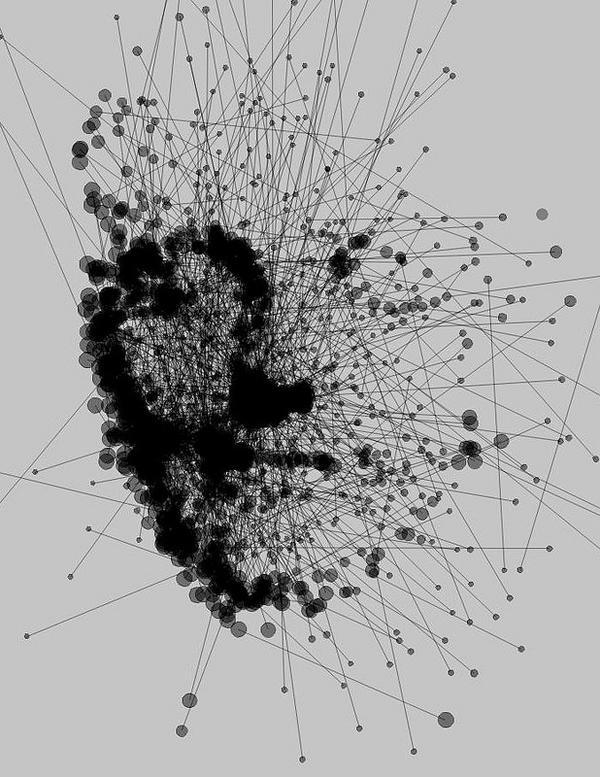#### Howdy, Stranger!

We are about to switch to a new forum software. Until then we have removed the registration on this forum.

# how to place dots and circles acquired from an image instead of random dots and circles?

I got this code:

``````Circle[] circles;
int numCircles = 50;
int maxDistance;

void setup() {
size(300, 300);
smooth();

// create an array and fill it with circles
circles = new Circle[numCircles];
for (int i=0; i< numCircles; i++) {
circles[i] = new Circle(random(width), random(height), random(20, 90));
}
}

void draw() {
// clear background
background(0, 0, 20);

// update and display the circles
for (int i=0; i< numCircles; i++) {
circles[i].update();
circles[i].display();
}

// define maximum distance
maxDistance = mouseX;

// look of the lines
stroke(255, 100);
strokeWeight(0.5);

for (int i=0; i< numCircles; i++) {
// compare circle to other circles
for (int j=i+1; j< numCircles; j++) {
// draw line if distance is below 'maxDistance'
if (dist(circles[i].x, circles[i].y, circles[j].x, circles[j].y) < maxDistance) {
line(circles[i].x, circles[i].y, circles[j].x, circles[j].y);
}
}
}
}

class Circle {
float x, y, dia;

Circle(float x, float y, float dia) {
this.x =x;
this.y =y;
this.dia = dia;
}

void update() {
// code for movement here
// this is just some random displacement
x = x + random(-1, 1);
y = y + random(-1, 1);
}

void display() {
// code for drawing the circles
noStroke();
fill(60, 60, 190, 60);
ellipse(x, y, dia, dia);
}
}
``````

that thoes this:this code draws circles and points connected by lines. the position of the circles are defined by specific coordinates. Is it possible that a background image (by its pixels brightness) can place the center of the circles?

maybe something like thatTagged:

• .

• I'm afraid I don't totally understand your question. could you reword it?

If you're trying to find the center of a series of points you can just take the average of their x and y values.

here's how to format code: https://forum.processing.org/two/discussion/8045/how-to-format-code-and-text#latest

• the code that I attached places the centers of circles at given coordinates. I want the centers of circles placed relatively to a pixel brightness from a given image.

Something like that: load an image--- place dots based on contrast of the image-- draw circles over the dots-- connect the circles.

• Ok I think I understand now.

pixels[] or get() should solve your problem: https://processing.org/reference/pixels.html https://processing.org/reference/get_.html

you can use these to calculate brightness for a pixel. Use your image.pixels if you don't want the whole canvas.

Avg the brightness over sections and you should have a good way of determining whether or not to place a circle there. It looks like you have the rest worked out.

• You could try something like this:

``````PImage img;

void setup() {
size(512, 256);
background(0);
for (int i = 0; i<=256; i++) {
stroke(i);
line(0, i, height, i);
}
noStroke();
fill(128);
ellipse(128, 32, 50, 50);
fill(64);
rect(64-25, 64, 50, 128);
fill(196);
rect(196-25, 64, 50, 128);

img = get(0, 0, 256, 256);
}

void draw() {
//background(0);
image(img, 0, 0);
translate(width/2, 0);
int count = 0;
while (count < 100) {
float px = random(width/2);
float py = random(height);
float b = brightness(img.get(int(px),int(py)));
float r = random(256);
if(r<=b){
count++;
fill(random(255),random(255),random(255),10);
ellipse(px,py,3,3);
}
}
}
``````
• let me rephrase my question: can anyone make a code that generate this image?• Yes.

``````String http = "https://";
size(img.width,img.height);
image(img,0,0);
``````

Now listen. There are two steps to doing this. The first step is to take the image you want it to look like and convert it to black and white. The second step is to use the brightness to randomly draw more circles/lines in a given area.

The second step I have shown you how to do.

The first step is simple: just call filter(GRAY); on your image.

Now since you know how to do both steps, DO IT YOURSELF.

If you still can't for some reason, ATTEMPT IT YOURSELF.

Then you can post the code of your attempt here to get more help. No, posting the example code you have does not count as an attempt. You actually have to try to understand what the example code is doing, and then use it as a basis to get the effect you want in your attempt.

• thank you.

• It's been years since I touched processing (probably back in 2012?), anyway, tutorials are tough becuase they are either too easy or skip some of the basics I need to revisit. I guess I just need to be patient.

This simple visual that certainly is overused (blockchain/neural networks :) but is still very cool, this visual of animated points with connected lines, is just so cool. The idea on this feed to sync it to an image is even cooler. Hoping I can inspire someone to share more code.

But also thought I'd share for BEGINNERS with ambition, the sources I have been using all day to try to get up to speed on this animation.

I'll save you a couple of hours, skipping the references and general examples and get right to what I found the most valuable tutorials:

Thanks for all the shared work! @360 Render did you finally solve your challenge?

• i dont know if it's too late but i do this work: https://www.openprocessing.org/sketch/548016

• This discussion is from Feb 2016.... why answer here...

code not by me but from the forum

``````// Create a second image (same size as test1.jpg) to act as a mask.
// This image has just 2 colours to determine where the dots can be placed. Then replace line 22 with the algorithm

//do {
//  calculate random x position (rx)
//  calculate random y position (ry)
//  get colour for [rx,ry] from mask image
//while(colour under [rx,ry] on mask image is in invalid area)
//dots[dotNo] = new PVector(rx, ry);

PVector[] dots;
color[] cols;

int numDots = 200; // !!!!!!!!!!!!!!!!!!!!!!!!!!!!!!!!!!!!!!
int dotNo = 0;

float maxRange = 50.0f;
float minRange = 20.0f;

static final String NAME = "test2", EXT = ".jpg";

PImage img ;

void setup() {
size(400, 400);
colorMode(RGB, 255);
background(255);
stroke(50, 20);
smooth();
myMask = createImage (img.width, img.height, RGB);

color blackColor= color(0);
for (int x=0; x < img.width; x++) {
for (int y=0; y < img.width; y++)
{
color thisColor = img.get(x, y);
//if (red(thisColor) > 200)
if (brightness(thisColor) > 166)  // > 100 or > 166 important !!!!
} // for
} // for

dots = new PVector[numDots];
cols = new color[numDots];
}

void draw() {
background(255);
if (dotNo < numDots) {
// dots[dotNo] = new PVector(random(img.width), random(img.height)); //line 22
int rx = int(random(img.width));
int ry = int(random(img.height));
if ( colormyMask != color(0) ) {
dots[dotNo] = new PVector(rx, ry);
cols[dotNo] =  color(img.get(rx, ry));
dotNo++;
} // if
}
renderLines();
}

void renderLines() {
for (int i=0; i<dotNo; i++) {
float dia = brightness(cols[i]);
println(dia);
dia=map(dia, 170, 255, 0, 33);
fill(0, 0, 255);
ellipse(dots[i].x, dots[i].y, dia, dia);
}

for (int i=0; i<dotNo; i++) {
for (int j=i+1; j<dotNo; j+=1) {
float d = dist(dots[j].x, dots[j].y, dots[i].x, dots[i].y);
if (d<maxRange &&
d>minRange) {
strokeWeight(20/d);
stroke( cols[i] );
line(dots[j].x, dots[j].y, dots[i].x, dots[i].y);
}
}
}
}
//
``````